### Day 12 - Operations with Radicals - 09.03.14

 Bell RingerGo over yesterday's Exit TicketIf you did not get a B or higher, I suggest doing some more practice problems and then re-assessing.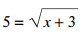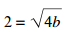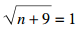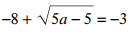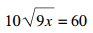ReviewExponent PropertiesProduct of PowersPower of a PowerPower of a ProductQuotient of PowersPower of a QuotientZero ExponentNegative ExponentsRadical PropertiesProduct of Square RootsQuotient of Square RootsRationalizing the DenominatorRational ExponentsExponential Growth and DecayLessonOperations with RadicalsExtended Practicepage 595 #5-9 (evens)page 595 #15-29 (evens)Radical EquationsExtended Practicepage 600 #5-13 (odds)page 601 #17-29 (odds)Exit Ticket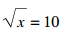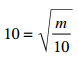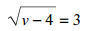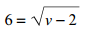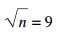Lesson Objective(s) How are exponent properties applied to simplify problems? How are radical properties used to simplify radical expressions and solve radical equations? Standard(s) N.RN.1  Explain how the definition of the meaning of rational exponents follows from extending the properties of integer exponents to those values, allowing for a notation for radicals in terms of rational exponents. N.RN.2  Rewrite expressions involving radicals and rational exponents using the properties of exponents. A.CED.1 Create equations and inequalities in one variable and use them to solve problems. Include equations arising from linear and quadratic functions, and exponential functions. Mathematical Practice(s) #2: Reason abstractly and quantitatively Students will use concrete examples of numerical manipulation to examine closure of rational and irrational numbers. For example, students will use numeric examples of sums and products of rational numbers to generalize the closure of rational numbers under addition and multiplication. #4: Model with mathematics Students will create equations using rational or radical expressions to represent mathematical models of real-world situations like interest rates or depreciation.